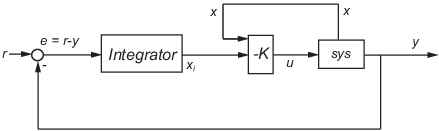Main Content

# lqi

Linear-Quadratic-Integral control

## Syntax

```[K,S,e] = lqi(SYS,Q,R,N) ```

## Description

`lqi` computes an optimal state-feedback control law for the tracking loop shown in the following figure.For a plant `sys` with the state-space equations (or their discrete counterpart):

`$\begin{array}{l}\frac{dx}{dt}=Ax+Bu\\ y=Cx+Du\end{array}$`

the state-feedback control is of the form

`$u=-K\left[x;{x}_{i}\right]$`

where xi is the integrator output. This control law ensures that the output y tracks the reference command r. For MIMO systems, the number of integrators equals the dimension of the output y.

`[K,S,e] = lqi(SYS,Q,R,N)` calculates the optimal gain matrix `K`, given a state-space model `SYS` for the plant and weighting matrices `Q`, `R`, `N`. The control law u = –Kz = –K[x;xi] minimizes the following cost functions (for r = 0)

• $J\left(u\right)={\int }_{0}^{\infty }\left\{{z}^{T}Qz+{u}^{T}Ru+2{z}^{T}Nu\right\}dt$ for continuous time

• $J\left(u\right)=\sum _{n=0}^{\infty }\left\{{z}^{T}Qz+{u}^{T}Ru+2{z}^{T}Nu\right\}$ for discrete time

In discrete time, `lqi` computes the integrator output xi using the forward Euler formula

`${x}_{i}\left[n+1\right]={x}_{i}\left[n\right]+Ts\left(r\left[n\right]-y\left[n\right]\right)$`

where Ts is the sample time of `SYS`.

When you omit the matrix `N`, `N` is set to 0. `lqi` also returns the solution `S` of the associated algebraic Riccati equation and the closed-loop eigenvalues `e`.

## Limitations

For the following state-space system with a plant with augmented integrator:

`$\begin{array}{l}\frac{\delta z}{\delta t}={A}_{a}z+{B}_{a}u\\ y={C}_{a}z+{D}_{a}u\end{array}$`

The problem data must satisfy:

• The pair (Aa,Ba) is stabilizable.

• R > 0 and $Q-N{R}^{-1}{N}^{T}\ge 0$.

• $\left(Q-N{R}^{-1}{N}^{T},{A}_{a}-{B}_{a}{R}^{-1}{N}^{T}\right)$ has no unobservable mode on the imaginary axis (or unit circle in discrete time).

## Tips

`lqi` supports descriptor models with nonsingular E. The output `S` of `lqi` is the solution of the Riccati equation for the equivalent explicit state-space model

`$\frac{dx}{dt}={E}^{-1}Ax+{E}^{-1}Bu$`

## References

 P. C. Young and J. C. Willems, “An approach to the linear multivariable servomechanism problem”, International Journal of Control, Volume 15, Issue 5, May 1972 , pages 961–979.

## See Also

Introduced in R2008b

## Support

#### Learn how to automatically tune PID controller gains

Download code examples